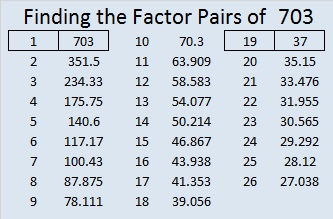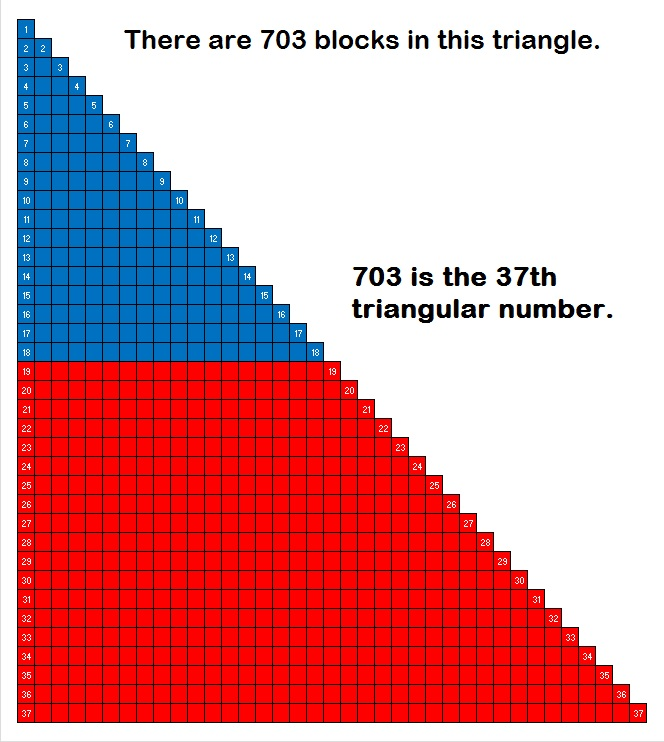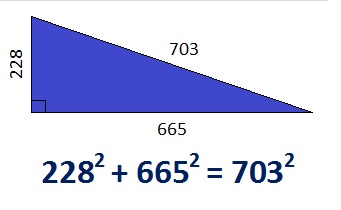# What Kind of Shape is 703 in?

• 703 is a composite number.
• Prime factorization: 703 = 19 x 37
• The exponents in the prime factorization are 1 and 1. Adding one to each and multiplying we get (1 + 1)(1 + 1) = 2 x 2 = 4. Therefore 703 has exactly 4 factors.
• Factors of 703: 1, 19, 37, 703
• Factor pairs: 703 = 1 x 703 or 19 x 37
• 703 has no square factors that allow its square root to be simplified. √703 ≈ 26.514147.703 is a fascinating number:As shown in that graphic, since the square of 703 can be broken apart and added back together to become itself again, 703 is the 7th Kaprekar number. Thank you OEIS.org for that fun fact.

What kind of shape is 703 in?

703 is the 37th triangular number:703 is a triangular number because 37(38)/2 = 703. If we move part of the triangle, those blocks can be neatly rearranged into this rectangle:About half of all triangular numbers are also hexagonal numbers. 703 is the 19th hexagonal number because (19⋅2)(19⋅2 – 1)/2 = 703.

In the past I found counting the dots in graphics of larger hexagonal numbers to be a nightmare, but yesterday I saw a small graphic for hexagonal number 28 that used different colors for each expansion. I challenged myself to make a graphic showing that 703 is a hexagonal number. I made it in excel using o’s in different colors. When I finished, I told excel to replace the o’s with •’s. Excel informed me that it made 703 replacements.  Then I adjusted the size of the cells to make the hexagon smaller. I also attempted to make the outer-most hexagon look as symmetrical as possible.Hexagonal numbers are not symmetrical the way hexagonal snowflakes are. I thought it would be cool to make 703 into a snowflake, but I didn’t succeed because 703 is just too big and it lacks symmetry. Nevertheless, I must share these directions to make snowflakes that are indeed 6-sided. (Using paper dinner napkins instead of regular paper makes folding and cutting them much simpler.)

703 is a palindrome in three different bases:

• 383 base 14; note that 3(196) + 8(14) + 3(1) = 703
• 111 base 26; note that 1(676) + 1(26) + 1(1) =703
• JJ base 36; note that J is equivalent to 19 in base 10 and 19(36) + 19(1) = 703

Since 37 is one of its factors, 703 is the hypotenuse of the Pythagorean triple we see in this triangle:If you divide each number in the triple by 19, you’ll get the primitive Pythagorean triple 12-35-37.

703 is also a leg in some Pythagorean triple triangles:

• 504-703-865 (a primitive generated from 2(28)(9), 28² – 9², 28² + 9²) Note that 28 + 9 = 37 and 28 – 9 = 19. Both 37 and 19 are prime factors of 703.
• 703-6660-6697
• 703-12996-13015
• 703-247104-247105 (another primitive)## 3 thoughts on “What Kind of Shape is 703 in?”

1.kelvinvoskuyl

Is 703 also the only hexagonal number to be the sum of two consecutive of them?

•ivasallay

Hexagonal number 703 is, indeed, the sum of two consecutive hexagonal numbers, 325 and 378. It may be the only hexagonal number that can make that claim, but I am not certain.

This site uses Akismet to reduce spam. Learn how your comment data is processed.